# Yield curve

The yield curve describes the interest rates an investor can earn by investing their money over different investment horizons. The yield curve thus covers the short-term, the medium-long term and the long-term investment horizon. The short-term interest rates are to a major extent controlled and influenced by central banks. The major central banks are the Federal Reserve (FED), the Bank of England (BoE), the European Central Bank (ECB) and the Bank of Japan (BoJ).

## Pure expectation theory: short and long-term rates

How the different interest rates over different investment horizons are related, can be explained using the ‘pure expectations’ theory. This theory states that investors can choose freely to either invest their money for a long period (e.g. for 2 years) at a certain interest rate or they can opt to invest their money over a combination of shorter periods (e.g. now for 1 year and next year again for 1 year). Because investors are supposed to be rational and don’t have a preference between different investment horizons, both investment strategies should yield the same return. If both strategies don’t yield the same return, then an arbitrage opportunity exists. Some investors will then exploit the profit opportunity, until the relationship holds again.

The formula below shows the basic relationship between short and long-term interest rates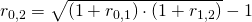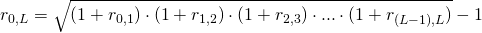Note that long-term interest rates are a geometric average of the short-term interest rates. As a consequence, changes in short-term interest rates will only have a limited impact on long-term interest rates. As a result, long-term interest rates are much more stable than short-term rates.

## Forward rate

Using the implications of the ‘pure expectations’ theory, we can calculate forward interest rates that can be used for discounting cash flows. Forward interest rates are simply future short-term interest rates that the market expects. We can determine this expected interest rate for investing money for one year, at the start of next year (r1,2) by using the interest rates we observe today. Today, we know the current 1-year short-term interest rate r0,1, as well as the current 2-year interest rate r0,2. We don’t observe r1,2, but we can infer it from the observed rates using the formula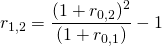## Liquidity premium theory: short and long-term rates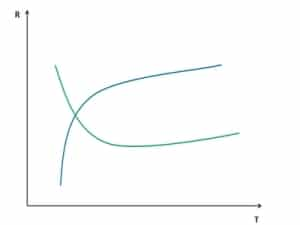In practice, the yield curve is almost always upward sloping. This means that long-term interest rates are generally higher than short-term rates most of the time. Therefore, short and long-term interest rates are not perfect substitutes. This is probably because different investors have a preference for different investment horizons when investing. However, as investing for longer periods of time means that the investor cannot withdraw his money for quite some time. He or she requires an additional compensation for long-term investments.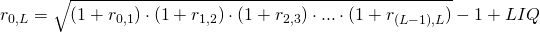## Summary

The yield curve describes the structure of interest rates over different investment horizons. Based on ‘pure expectations theory’, it is possible to calculate forward rates. These can be used to discount cash flows.